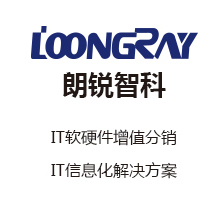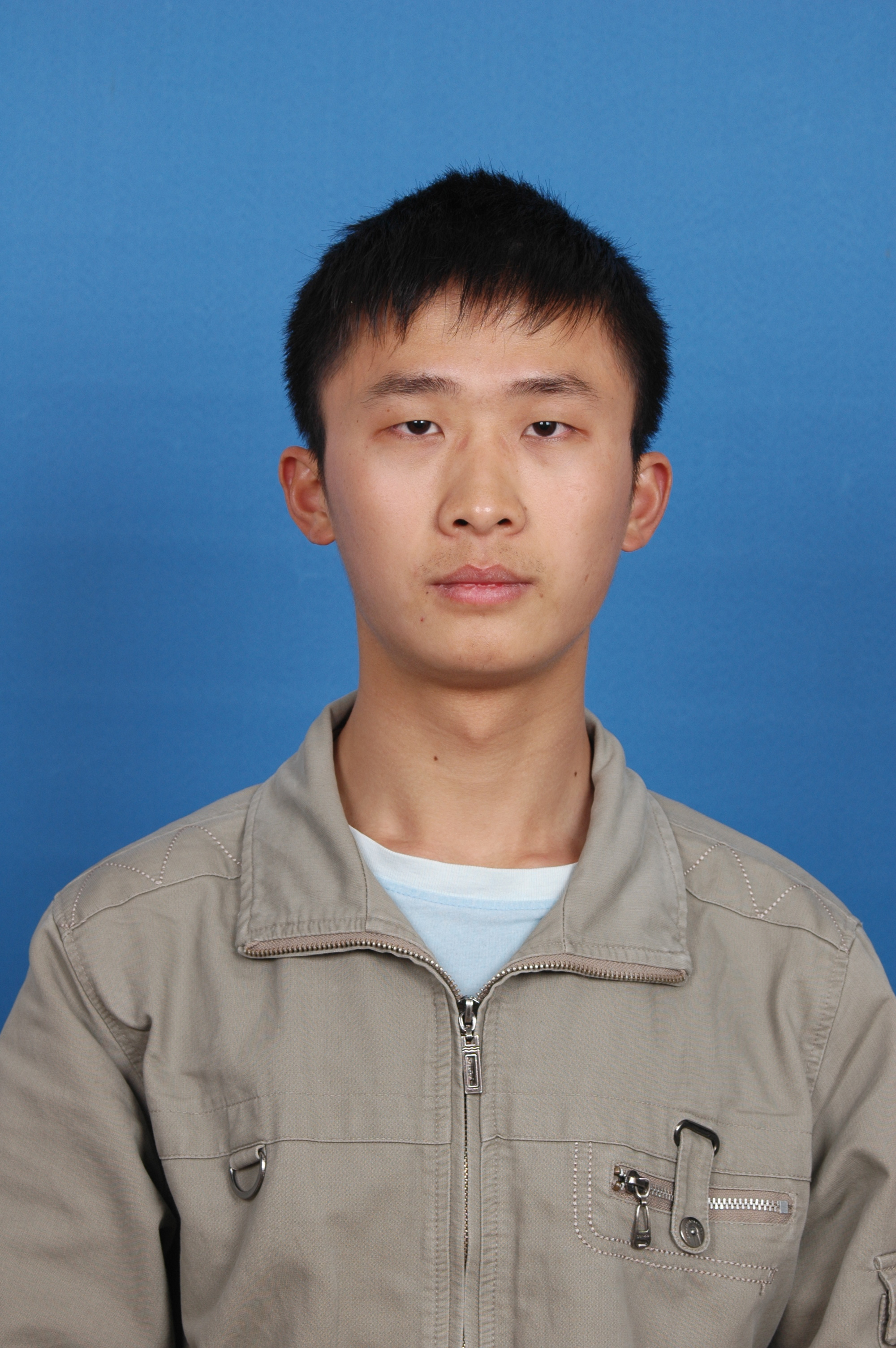# 【嵌入式开发】C语言 内存分配 地址 指针 数组 参数 实例解析（二）

+关注继续查看

3. 指针与地址

(1) & 与 * 操作

-- 表达式解析 : 将 c 的地址赋值给 变量 p, p 是指向 c 变量的指针;

-- & 可以使用的情况 : 取地址操作 只能用于内存中的对象, 如变量 或 数组, 栈内存 堆内存 都可以;

-- & 不适用的情况 : 不能用于 表达式, 常量, register类型变量;

-- 声明指针 : int *p ; 该表达式的含义是 *p 的结果是 int 类型, 声明变量 a, int a, 声明指针 *p , int *p;

-- 获取指针指向的值 : int a = *p ;

(2) 指针定义解析

-- 原理 : *p 和 max()返回值 类型都是 int 类型;

-- 例外 : void *p 可以指向任何类型, 但是 p 不能进行取值运算, *p 是错误的, 因为不知道 p 指向的数据类型;

(3) 指针运算及示例

-- 算数运算 : x = x + 1; 等价于 *p = *p + 1 ; int y = x + 1; 等价于 int y = *p + 1;

-- 自增运算 : 前提 : ++, * 运算顺序是自右向左;  ++*p 和 (*p)++, p 指向的值自增1, 注意要加上括号, 否则会将地址自增;

-- 指针赋值 : int *p, *q; int a = 0; p = &a; q = p; 最终结果 p 和 q 都指向了 变量 a;

/*************************************************************************
> Author: octopus
> Mail: octopus_work.163.com
> Created Time: Mon 10 Mar 2014 09:52:01 PM CST
************************************************************************/
#include<stdio.h>
int main(int argc, char ** argv)
{
int *p, *q;
int a = 10, b;
//p指针指向a变量
p = &a;
//*p 可以代替 a 进行运算
++*p;
b = *p + 5;
//指针之间可以直接相互赋值
q = p;
//打印 p 和 q 指针指向的值
printf("*p = %d \n", *p);
printf("*q = %d \n", *q);
return 0;
}

[root@ip28 pointer]# gcc pointer_address.c
[root@ip28 pointer]# ./a.out
*p = 11
*q = 11

4. 函数参数的传值调用和传址调用

(1) 传值调用 和 传址调用

/*************************************************************************
> File Name: swap.c
> Author: octopus
> Mail: octopus_work.163.com
> Created Time: Mon 10 Mar 2014 11:07:18 PM CST
************************************************************************/
#include<stdio.h>
void swap_1(int a, int b)
{
int temp;
temp = a;
a = b;
b = temp;
printf("swap_1 传值 函数 a = %d, b = %d \n", a, b);
}
void swap_2(int *a, int *b)
{
int temp;
temp = *a;
*a = *b;
*b = temp;
printf("swap_2 传址 函数 a = %d, b = %d\n", *a, *b);
}
int main(int argc, char **argv)
{
int a = 10, b = 5;
printf("初始值 : a = %d, b = %d \n\n", a, b);
swap_1(a, b);
printf("执行 swap_1 函数, a = %d, b = %d \n\n", a, b);
swap_2(&a, &b);
printf("执行 swap_2 函数, a = %d, b = %d \n", a, b);
return 0;
}

[root@ip28 pointer]# gcc swap.c
[root@ip28 pointer]# ./a.out

swap_1 传值 函数 a = 5, b = 10

swap_2 传址 函数 a = 5, b = 10

-- 传值调用 : swap_1 是传值调用, 传入的是 main 函数中的 a b 两个变量的副本, 因此函数执行完毕后, 主函数中的值是不变的;

-- 传址调用 : swap_2 是传址调用, 传入的是 a , b 两个变量的地址 &a, &b, 当在swap_2 中进行修改的时候, 主函数中的 a,b变量也会发生改变;

(2) 高级示例

getch 和 ungetch 函数 :

-- 使用场景 : 当进行输入的时候, 不能确定是否已经输入足够的字符, 需要读取下一个字符, 进行判断, 如果多读取了一个字符, 就需要将这个字符退回去;

-- 使用效果 : getch() 和 ungetch() 分别是预读下一个字符, 和 将预读的字符退回去, 这样对于其它代码而言, 没有任何影响;

/*************************************************************************
> File Name: getint.c
> Author: octopus
> Mail: octopus_work.163.com
> Created Time: Mon 10 Mar 2014 11:40:19 PM CST
************************************************************************/
#include <stdio.h>
#include <stdlib.h>
#include <ctype.h>
#define SIZE 5
int getint(int *p)
{
//sign 是用来控制数字的正负
int c, sign;
//跳过空白字符, 如果是空白字符, 就会进行下一次循环, 直到不是空白字符为止
while(isspace(c = getc(stdin)));
//如果输入的字符不是数字, 就将预读的数据退回到标准输入流中
if(!isdigit(c) && c != EOF && c != '+' && c != '-')
{
ungetc(c, stdin);
return 0;
}
/*
* 如果预读的是减号, 那么sign 标识就是 -1,
* 如果预读的是加号, 那么sign 标识就是 1;
*/
sign = (c == '-') ? -1 : 1;
//如果 c 是 加号 或者 减号, 再预读一个字符&
if(c == '+' || c == '-')
c = getc(stdin);
for(*p = 0; isdigit(c); c = getc(stdin))
*p = 10 * *p + (c - '0');
*p *= sign;
if(c != EOF)
ungetc(c, stdin);
return c;
}
int main(int argc, char **argv)
{
int n, array[SIZE], i;
for(n = 0; n < SIZE && getint(&array[n]) != EOF; n++);
for(i = 0; i < SIZE; i++)
{
printf("array[%d] = %d \n", i, array[i]);
}
return 0;
}

octopus@octopus-Vostro-270s:~/code/c/pointer$./a.out 123 123 43 674 1 array = 123 array = 123 array = 43 array = 674 array = 1 5. 指针 和 数组 指针数组比较 : -- 可互相替代 : 数组下标执行的操作都可以使用指针替代; -- 效率比较 : 使用指针操作效率比数组要高; 指针 与 数组初始化 : -- 声明数组 : int a; 定义一个长度为10 的int数组; -- 声明指针 : int *p; 定义一个指针, 该指针指向整型; -- 相互赋值 : p = &a, 将数组第一个元素的地址赋值给指针变量; -- 使用指针获取数组对象 : *p 等价于 a, *(p + 1) 等价于 a, *(p + i)等价于 a[i]; -- 注意地址的运算 : p + i , 在地址运算上, 每次增加 sizeof(int) * i 个字节; 将数组赋值给指针的途径 : -- 将数组第一个元素地址赋值给指针变量 : p = &a; -- 将数组地址赋值给指针变量 : p = a; 指针 和 数组 访问方式互换 : 前提 int *p, a; p = a; -- 数组计算方式 : 计算a[i]的时候, 先将数组转化为 *(a + i)指针, 然后计算该指针值; -- 取值等价 : a[i] 等价于 *(p + i); -- 地址等价 : &a[i] 与 a + i 是等价的; -- 指针下标访问 : p[i] 等价于 *(p + i); -- 结论 : 通过数组和下标 实现的操作 都可以使用 指针和偏移量进行等价替换; 指针 和 数组 的不同点 : -- 指针是变量 : int *p, a; p = a 和 p++ 没有错误; -- 数组名不是变量 : int *p, a; a = p 和 a++ 会报错; 数组参数 : -- 形参指针 : 将数组传作为参数传递给函数的时候, 传递的是数组的首地址, 传递地址, 形参是指针; 数组参数示例 : -- 函数参数是数组 : 函数传入一个字符串数组参数, 返回这个字符串长度; /************************************************************************* > File Name: array_param.c > Author: octopus > Mail: octopus_work.163.com > Created Time: Sat 15 Mar 2014 12:46:57 AM CST ************************************************************************/ #include<stdio.h> //计算字符串长度 int strlen(char *s) { int n; for(n = 0; *s != '\0'; s++) n++; return n; } int main(int argc, char** argv) { printf("strlen(djdhaj) = %d \n", strlen("djdhaj")); printf("strlen(12) = %d \n", strlen("12")); printf("strlen(dfe) = %d \n", strlen("dfe")); } -- 执行结果 : warning: conflicting types for built-in function ‘strlen’, 原因是 C语言中已经有了 strlen 函数了, 如果改一个函数名, 就不会有这个警告了; [root@ip28 pointer]# gcc array_param.c array_param.c:12: warning: conflicting types for built-in function ‘strlen’ [root@ip28 pointer]# ./a.out strlen(djdhaj) = 6 strlen(12) = 2 strlen(dfe) = 3 数组和指针参数 : 将数组名传给参数, 函数根据情况判断是作为数组还是作为指针; -- 实参 : 指针偏移量 和 数组下标 都可以作为 数组或指针函数形参, 如 数组情况fun(&array) 或者 指针情况fun(p + 2); -- 形参 : 函数的形参可以声明为 fun(int array[]), 或者 fun(int *array), 如果传入的是数组的第二个元素的地址, 可以使用array[-2]来获数组取第一个元素; 数组指针参数示例 : /************************************************************************* > File Name: param_array_pointer.c > Author: octopus > Mail: octopus_work.163.com > Created Time: Sat 15 Mar 2014 01:28:33 AM CST ************************************************************************/ #include<stdio.h> //使用指针做形参 取指针的前两位 和 当前位 void fun_p(int *p) { printf("*(p - 2) = %d \n", *(p - 2)); printf("*p = %d \n", *p); } //使用数组做形参 取数组的 第-2个元素 和 第0个元素 void fun_a(int p[]) { printf("p[-2] = %d \n", p[-2]); printf("p = %d \n", p); } int main(int argc, char **argv) { int array[] = {1,2,3,4,5}; //向指针参数函数中传入指针 printf("fun_p(array + 2) : \n"); fun_p(array + 2); //向数组参数函数中传入数组元素地址 printf("fun_a(&array) : \n"); fun_a(&array); //向指针参数函数中传入数组元素地址 printf("fun_p(&array) : \n"); fun_p(&array); //向数组参数函数中传入指针 printf("fun_a(array + 2) : \n"); fun_a(array + 2); return 0; } 执行效果 : [root@ip28 pointer]# gcc param_array_pointer.c [root@ip28 pointer]# ./a.out fun_p(array + 2) : *(p - 2) = 1 *p = 3 fun_a(&array) : p[-2] = 1 p = 3 fun_p(&array) : *(p - 2) = 1 *p = 3 fun_a(array + 2) : p[-2] = 1 p = 3 版权声明：本文内容由阿里云实名注册用户自发贡献，版权归原作者所有，阿里云开发者社区不拥有其著作权，亦不承担相应法律责任。具体规则请查看《阿里云开发者社区用户服务协议》和《阿里云开发者社区知识产权保护指引》。如果您发现本社区中有涉嫌抄袭的内容，填写侵权投诉表单进行举报，一经查实，本社区将立刻删除涉嫌侵权内容。 作者高分内容 更多 相关文章2018年嵌入式开发前景分析 1159 0[裴礼文数学分析中的典型问题与方法习题参考解答]5.1.2 设$\sed{a_n}$为等差数列,$a_{n+1}-a_n=d>0\ (n=1,2,\cdots)$,$m$为一正整数. 计算 $$\bex S=\vsm{n}\frac{1}{a_n\cdot a_{n+1}\cdots a_{n+m}}. 725 0[裴礼文数学分析中的典型问题与方法习题参考解答]5.1.1 设 k,i,j 都是自然数, 且 k=i+j, 试求级数 \dps{\vsm{n}\frac{1}{(kn-i)(kn+j)}} 的和. 解答: 原级数的前 N 项和为$$\beex \bea \sum_{n=1}^N \frac{1}{(kn-i)(kn+j)} &=\f... 790 0[裴礼文数学分析中的典型问题与方法习题参考解答]5.1.4 证明: 当$p\geq1$时, $$\bex \vsm{n}\frac{1}{(n+1)\sqrt[p]{n}} 501 0[裴礼文数学分析中的典型问题与方法习题参考解答]4.5.16 例 4.5.37 的逆命题不成立, 即 f(x) 在 (0,1) 内单调, \dps{\vlm{n}\frac{1}{n}\sum_{i=1}^{n-1} f\sex{\frac{i}{n}}} 存在, \dps{\int_0^1 f(x)\rd x} 可以不收敛. 581 0嵌入式工业主板定制选型要点分析 主板是电脑的核心部件,无论是商用的还是工业级的, 电脑就是通过主板将CPU等各种器件和外部设备有机地结合起来，形成一套完整的系统，因此电脑的整体运行速度和稳定性在相当程度上取决于主板的性能。工业主板由于其应用范围更广泛，使用环境更复杂，因此用户对其功能、兼容性、可靠性要求更高。 1709 0[裴礼文数学分析中的典型问题与方法习题参考解答]4.5.12 f(x) 在 [a,+\infty) 上连续且 \dps{\int_a^{+\infty}f(x)\rd x} 收敛. 问能否断定: \exists\ x_n\to\infty, 使 \dps{\vlm{x}f(x_n)=0}? 为什么? (南开大学) 解答: 肯定. 499 0[裴礼文数学分析中的典型问题与方法习题参考解答]4.5.13 设 f(x) 于任一有限区间 [0,a]\ (a>0) 上正常可积, 于 [0,\infty) 上绝对可积, 则$$\bex \vlm{n}\int_0^\infty f(x)|\sin nx|\rd x =\frac{2}{\pi}\int_0^\infty f(x)\rd x. 620 0[裴礼文数学分析中的典型问题与方法习题参考解答]4.5.11 设$f(x)$是$0\leq x
533 0[裴礼文数学分析中的典型问题与方法习题参考解答]4.5.3

480 0

2605

0

OceanBase 入门到实战教程